# MCAT Physical : Scalar and Vector Quantities

## Example Questions

### Example Question #1 : Scalar And Vector Quantities

A student measures the mass, volume, weight, and density of a solid in SI units. Which measurement(s) has/have units that are not a type of derived unit?

Mass and weight

Weight and density

Mass and volume

Only mass

Only mass

Explanation:

There are two types of units: base units and derived units. Base units are a set of units from which all other units are derived, whereas derived units are the units derived from base units.

There are six main base units: meters, kilogram, second, Ampere, Kelvin, moles, and candela (unit for luminous intensity). The rest of the units are classified as derived units and will contain a combination of these base units. The SI units for the four measurements listed in question are as follows:

Mass: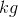Volume: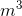(Liters)

Weight: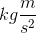(Newtons)

Density: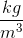This means that volume, weight, and density are derived units, whereas mass is a base unit.

### Example Question #2 : Scalar And Vector Quantities

Which of the following is true about SI derived units and base units?

There are more base units than derived units

Hours are a type of SI base unit

Units for force can be either derived units or base units

Derived units are obtained by adding, subtracting, multiplying, and/or dividing base units

Hours are a type of SI base unit

Explanation:

Derived units are derived from the six base units. A derived unit usually contains multiple base units that are combined by using multiplication and/or division; addition and subtraction of base units is not performed to obtain derived units.

The SI unit for force is Newtons. Newtons written in base units is; therefore, units for force is always a derived unit. A unit can be either a derived unit or a base unit, but it can never be both. Recall that there are only six base units and numerous derived units; therefore, there are more derived units than base units.

The SI base unit for time is seconds. Hours are derived by multiplying seconds by sixty. Hours are not a base unit.

### Example Question #3 : Scalar And Vector Quantities

The unit for mass is a type of __________ unit. The unit "moles" is a type of __________ unit.

base . . . base

base . . . derived

derived . . . derived

derived . . . base

base . . . base

Explanation:

Recall that the mass of a substance is usually measured in kilograms (), which is a type of base unit. Moles (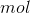) is a unit used to measure the amount of substance. Moles are also a type of base unit; therefore, both the unit for mass () and the unit for amount of substance () are measures of base units.

### Example Question #1 : Linear Motion

Which answer choice below includes only scalar quantities?

Velocity, displacement, force

Acceleration, energy, displacement

Distance, speed, time

Force, time, velocity

Displacement, time, acceleration

Distance, speed, time

Explanation:

Scalar quantities are those that can be described with magnitude only, as opposed to vectors, which include both magnitude and direction components. Distance, speed, and time are all scalars. Displacement is not a scalar, as it involves both the distance and the direction moved from a starting point. Velocity also includes a direction component, and is therefore a vector quantity.

### Example Question #4 : Scalar And Vector Quantities

An airplane is in flight. Which of the following is an example of a vector?

The speed of the plane

The angle at which the plane flies

None of these are vectors

The velocity of the plane

The distance traveled by the plane